### Home > GC > Chapter 5 > Lesson 5.1.4 > Problem5-36

5-36.

Which of the triangles below are similar to $ΔLMN$ at right? How do you know? Explain.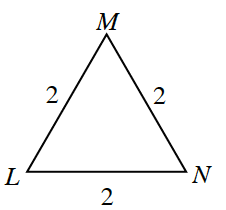1.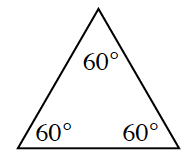1.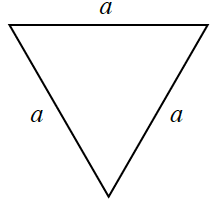1.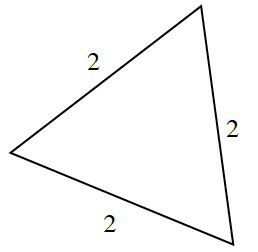1.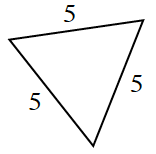Notice that $ΔLMN$ is an equilateral triangle. That means all sides and angles are equal in measure.

Triangles (a), (b), (c), and (d) are all similar because they are all equilateral triangles.
All three sides are proportional ((b), (c), and (d)) and $2$ sets of angles are equal in triangle (a).